The reader must excuse my bad english.

# Minesweeper is NP-complete

2014-08-13: I found this article [source] that shows that 1-minesweeper is also NP-complete.

## Introduction

In an article of the Symbolic Journal of Computation, Richard Kaye proposed a translation from a propositional logic formula into a minesweeper configuration, such as asking the question 'is there a solution to this formula ?' is the same as asking 'is this minesweeper configuration possible ?'. Because the first problem is NP-complete, and because the translation is in polynomial time, you have that minesweeper is NP-complete. See Richard Kaye's Minesweeper Pages for all the details. Unfortunately, his paper does not exist in electronic format, so you will have to go to your favorite library to read it.

Here, we simply propose a program implementing this translation. The program is far from efficient. Anyway, translating a logical formula into a minesweeper configuration is a bit of madness, because the minesweeper configuration is much bigger than the formula, and solving this configuration instead of the formula would be the act of some crazy mind. The translation is polynomial, but the resulting configuration is too big and simply cannot be handled.

For example, I have a formula saved in a 6,060 bytes long file, and my program produces a 2,099,200,686 bytes long file, representing the corresponding minesweeper configuration. A computer can handle a 6,060 bytes long file (a program called zchaff can solve the problem in less than 1 second). I know no program solving a minesweeper configuration able to handle a 2,099,200,686 bytes long file (by the way I know no program solving a minesweeper configuration). And this formula is just the translation of the question 'is there is number of 2 binary digits with the first one = 1 that divides 6 ?'. I have a program (available here) that can produce formulas for this question but with much bigger numbers than 6 and with more than 2 binary digits. I didn't even try to transform the produced formulas into minesweeper configurations. (I must admit that this program is far from generating the smallest formulas though.)

In the following, we first will show how to transform a formula into a minewseeper's configuration, such as Richard Kaye defined it. Then, we will see what are the basic blocks of minesweeper configurations that we use. Then, we will talk a bit about the programs we did.

## From a formula to a minesweeper configuration

In this section, we will take as an example the formula `(p or q) and (r or (not q))`, where `p`, `q` and `r` are boolean variables.

To translate this formula into a minesweeper configuration, we simply draw it, as illutrated by figure 1.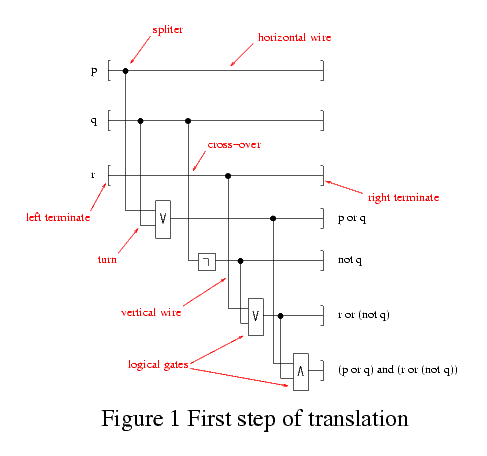This is a visual interpretation of the formula. We may think of it as being a hardware circuit, coding the formula. The tree variables come from the left, and go to the right. Then, each line represents a sub-formula of the main formula, which is simply the output of the last line.

From this visual interpretation, we derivate the corresponding minesweeper configuration. For this particular example, our program would give us a 2160x2784 image that the curious can see here (64,593 bytes). The figure 2 is a 25% reduction of it. (Note : I produced an image for the 6,060 bytes long example file, leading to a 1,193,161,327 bytes long png file, produced after several hours of calculation.)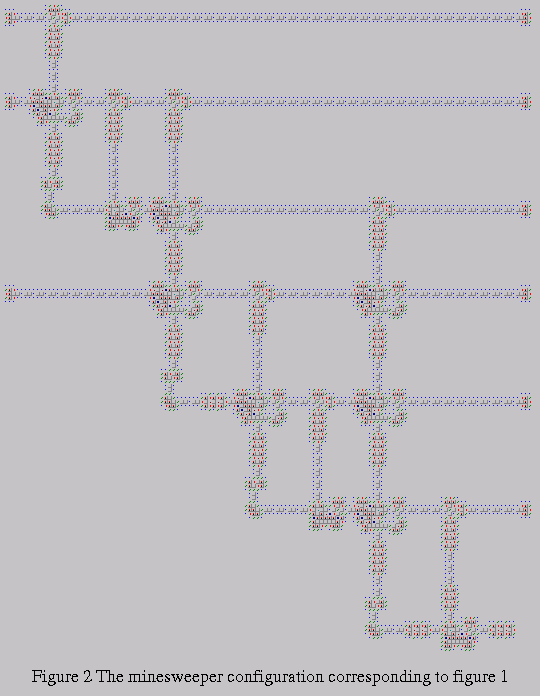Note that, due to the way our algorithm works, the lines are a bit interleaved, so `q` is not followed by `r`, as in figure 1, but directly by `p or q`, only then comes `r`. It would be easy to rewrite the code to get the wanted order.

The creation of the layout is very simple. The translation from this layout into a minesweeper configuration is straightforward. We won't describe the algorithms further. I could refer the interested reader to the source code, but it's a bit unclean. Try it anyway.

As we can see in figure 1, we have several basic blocks. Those are :

• wires,
• spliters,
• cross-over,
• terminates,
• turns,
• logical gates.
In the following section, we will give the corresponding minesweeper configuration to each of these elements. We won't enter too much into details. The interested reader is suggested to report to the original paper.

## Basic blocks

Here come descriptions of all the basic blocks.

First of all, one has to note that our grid has a 3x3 basis, meaning the smallest unit considered is not the cell but a square of cells of size 3x3. Some basic blocks created by Richard Kaye (and others) don't fit on this 3x3 basis, so we need to add some more cells to them to fit to this basis. This explains why if you look at some of ours blocks, they are bigger than the corresponding one introduced by Richard Kaye. This approach was choosen because the algorithms are simple with it.

### Wires

We have two kinds of wires : horizontal ones (from left to right) and vertical ones (from top to bottom).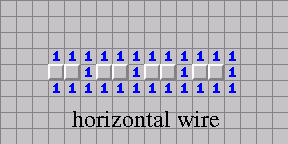The image above shows 4 blocks of horizontal wires.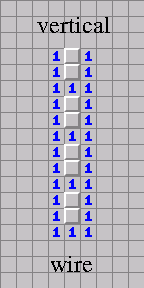The image above shows 4 blocks of vertical wires.

### Spliters

We have one kind of spliter. It will split a wire coming from the left into two wires, one going down and the other going right.The image above shows a spliter.

### Cross-over

Here, we also have one kind of cross-over. It will cross two wires, one coming from left and going to right and the other one coming from top and going to bottom.The image above shows a cross-over.

### Terminates

Terminates may be hard to understand if you don't know how all this works. I highly suggest you to read Richard Kaye's Minesweeper Pages to get it all.

Here we have two types of terminates. A left one and a right one.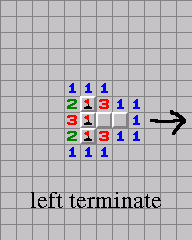The image above shows a left terminate.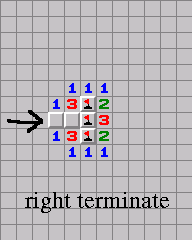The image above shows a right terminate.

### Turns

We have one type of turn. It turns a wire going from top to bottom into a wire going from left to right. It is a bit different from the one seen in Richard Kaye's paper because we have to handle the 3x3 alignment.The image above shows a turn.

### Logical gates

We can imagine a lot of logical gates. But we know that all the propositional logic can be expressed with only `not` and `or`, so we only have implemented those two gates, as basic blocks.

We have two `not` gates. One vertical and one horizontal.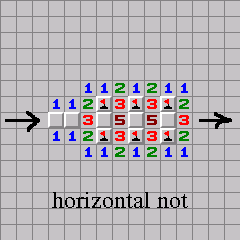The image above shows a horizontal not.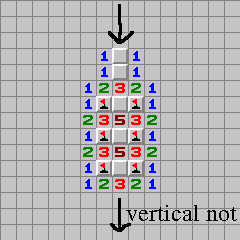The image above shows a vertical not.

We have one kind of `or`.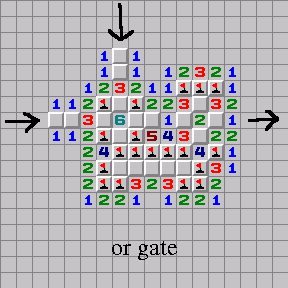The image above shows an or.

This basic `or` cannot be used as is (the same remark can be applied to the previous `not` gates as well), because wires, in our algorithm, come from top when arriving to the logical gates, so we have to create a gate taking two vertical wires as input, thus leading to the next `or` gate, where we added a turn (and the two vertical wires must not be too close, so this explains why we have some horizontal wires between the turn and the or). Now, this `or` can be seen as a box with two inputs and a output. We could have considered only a basic `or`, but placing all the blocks together would have been a much greater pain. With this box model, we only handle rectangles, much easier to deal with. Our algorithms are very simplified with this method.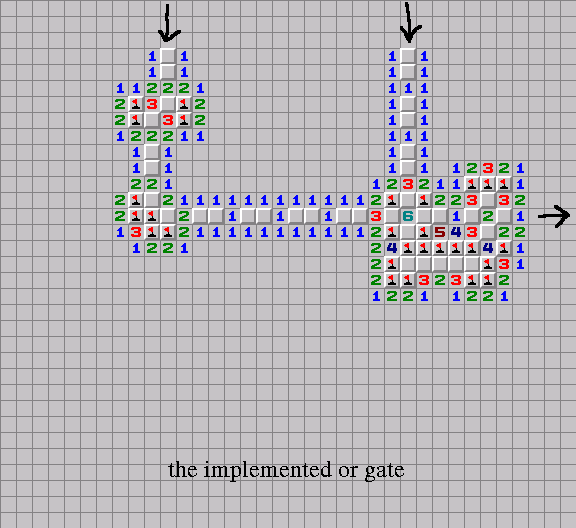The image above shows the implemented or.

By using both `not` and `or`, we can, as previously stated, express any formula. For example, we know that `a and b` has the same truth value for any `a` and `b` as `not ((not a) or (not b))`. Here comes this `and` gate we implemented.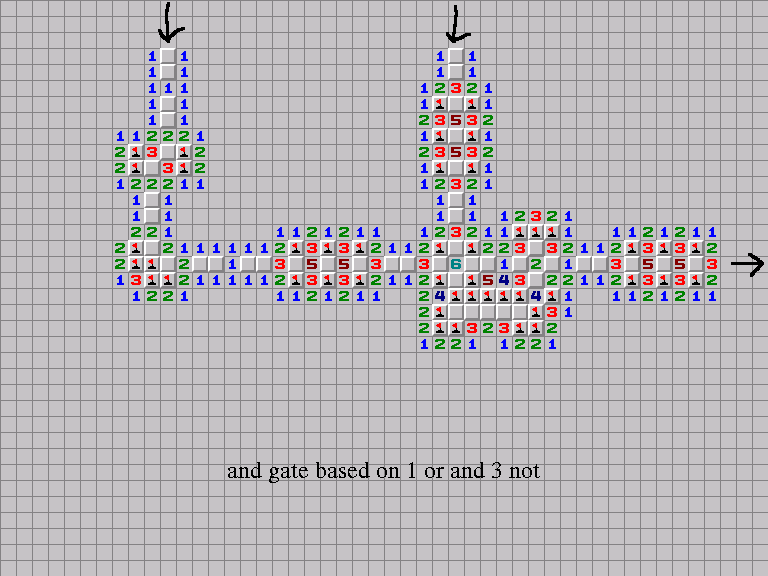The image above shows an and.

It's getting big.

So, those were the basic blocks.

## Programs

mines-1.0.1.tar.gz (released 2014-04-02) is the same as the 1.0.0, except it compiles with modern gcc.

mines-1.0.0.tar.gz (39,913 bytes) contains a program that will produce a minesweeper configuration, coming from a formula.

The formula has to be written in a conjonctive normal form (CNF), which is something like ```(a or b or (not c)) and (c or (not d)) and ((not a) or e or g or (not f))```. It is possible to convert any formula into something that has this form. But, in general, you will have an exponential blow-up. There exists technics to avoid this blow-up. The interested reader might consult for example On Generating Small Clause Normal Forms by Nonnengart, Roch and Weidenbach to get some ideas about this topic, but it is a bit tricky. Symbolic Model Checking using SAT procedures instead of BDDs is not primarily interested by this specific matter, but they recall a simple algorithm, easy to understand to transform a formula into CNF (the original paper dealing with this is not available online).

The file-format that is accepted by the program is the one of DIMACS, restricted to the CNF part of it.

There is a program included in the archive that generates CNF files, which correspond to a factorization problem. But those generated examples are really too big, as stated in the introduction. (Anyway, any realistic example of formula is probably too big to be translated into an usable minesweeper configuration.)

To build those programs, you will need a unix machine, a C compiler (gcc is fine). You will need libpng to be able to create png files.

Once downloaded, untar the archive, then read README. If there is a problem, contact me. The README says you need libgmp. This is wrong, sorry.

All the interesting code is in `main.c` and `main2.c`. Unfortunately, it is not very well documented, I apologize for that. If you want some better technical details, try to contact me.

Create surely before: Sat Aug 10 22:41:41 CEST 2002

Last update: Wed, 13 Aug 2014 11:55:00 +0200

Contact me at sed@free.fr.

You can check my main page if you feel it.